Lifting weights takes power but this quiz tests what you know about powers (note the 's').

# Powers (H)

Powers, indices, exponents – they all mean the same thing, and are a shorthand way to show that a number has been multiplied by itself. This GCSE Maths quiz will test your understanding of working with powers, both in numerical and algebraic formats.

If I multiply 3 by itself 4 times, it would be time-consuming to write this out using multiplication signs. Using a superscript ‘4’ is a nice, neat shorthand way to indicate this. In this example, ‘3’ is the base number and ‘4’ is the index, or power.

Once you start carrying out calculations involving powers, you soon spot little shortcuts in working out the size of the new power. These have evolved to become the Laws, or Rules, of Indices. They apply equally to numbers and algebra, and so it is vital that you know and can apply all the laws correctly as they can crop up in all areas of maths!

The multiplying and dividing rules are easily remembered, and if you forget the ‘power of a power’ just write it out in full and the rule should become obvious. The ones that catch people out are the roots, and forgetting how to convert between a negative index and a fraction with a positive index. If you don’t know what I am talking about, best you revise it!

Don’t forget the rules only work when the numbers, or algebraic terms, have the same base. If they don’t, you will have to work out the size of the actual numbers before you can complete the calculation.

Question 1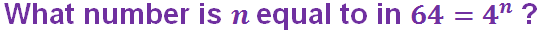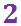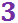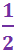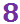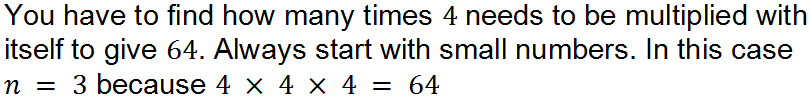Question 2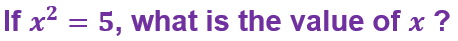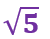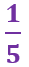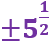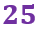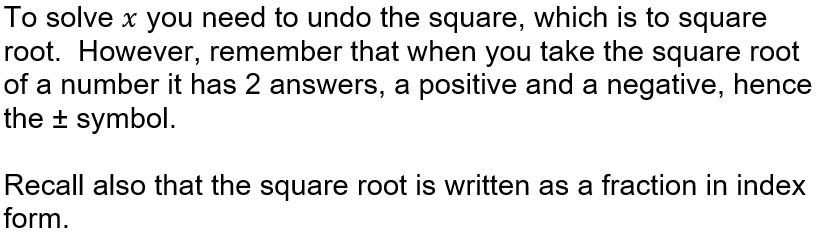Question 3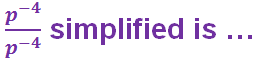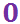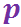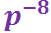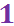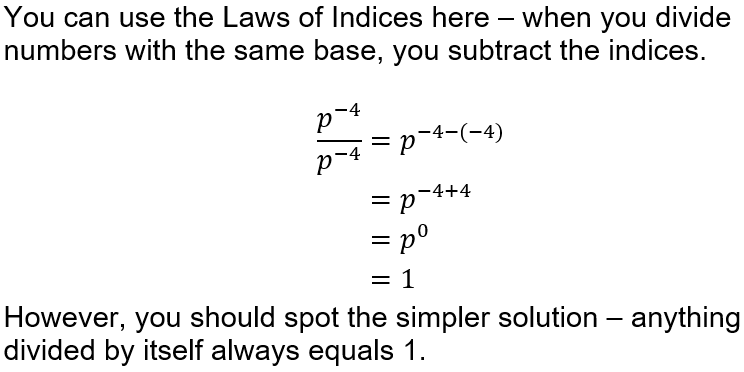Question 4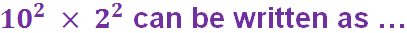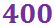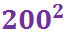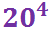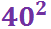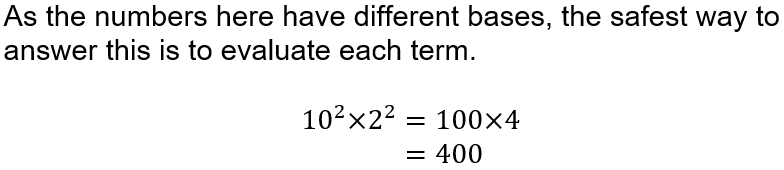Question 5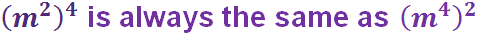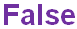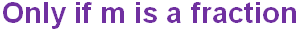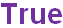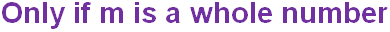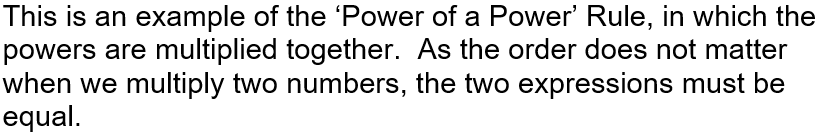Question 6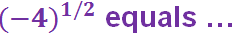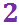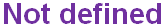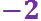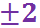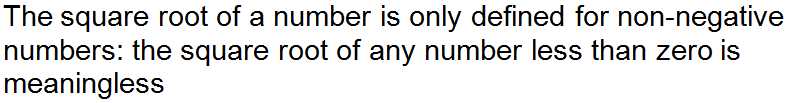Question 7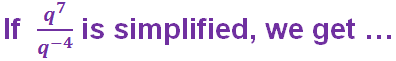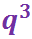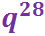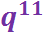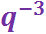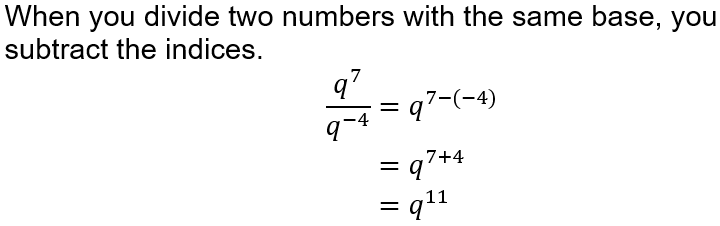Question 8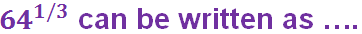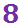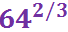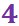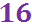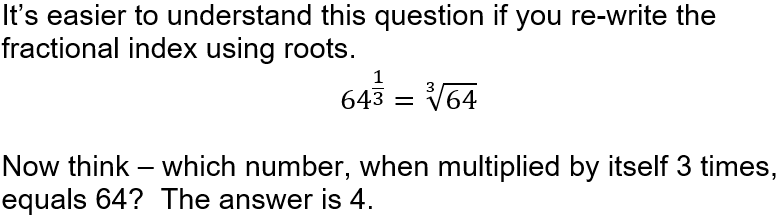Question 9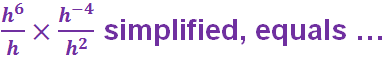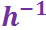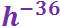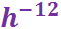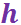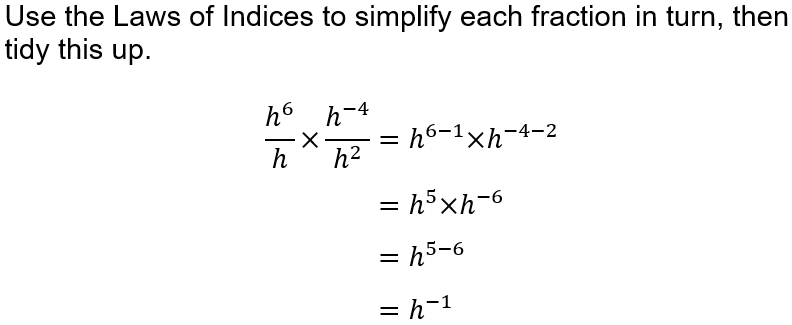Question 10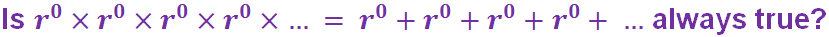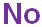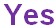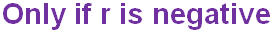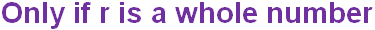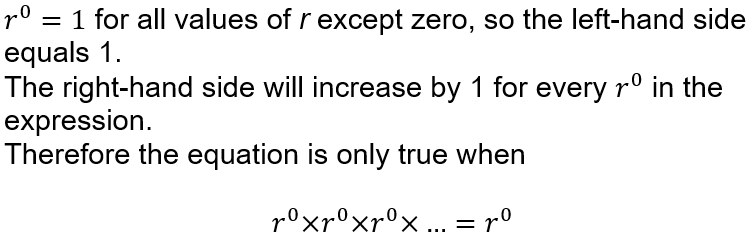Author:  Sally Thompson